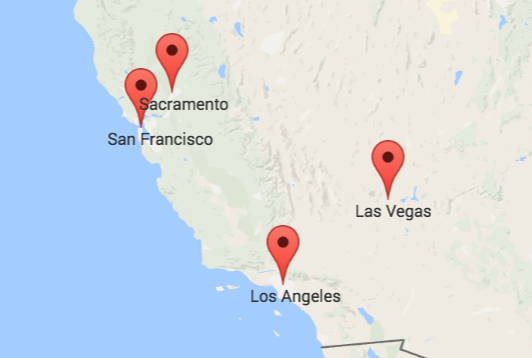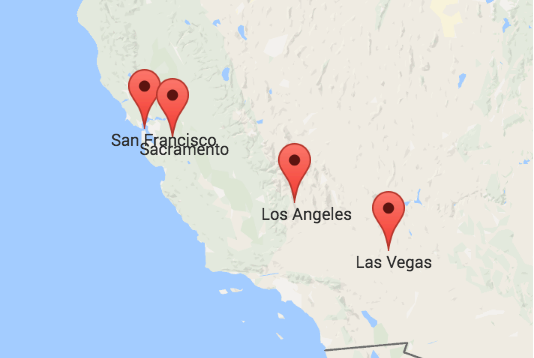# PyTorch for TensorFlow Users - A Minimal Diff

##### 07 March 2021

This is a migration guide for TensorFlow users that already know how neural networks work and what a tensor is. I have been using TensorFlow since 2016, but I switched to PyTorch in 2020. Although the key concepts of both frameworks are pretty similar, especially since TF v2, I wanted to make sure that I use PyTorch's API properly and don't overlook some critical difference. Therefore, I read through the currently listed beginner-level PyTorch tutorials, the 14 notes in the PyTorch documentation (as of version 1.8.0), the top-level pages of the Python API like torch.Tensor and torch.distributions, and some intermediate tutorials. For each tutorial and documentation page, I list the insights that I consider relevant for TensorFlow users.

# Convolutions in Autoregressive Neural Networks

##### 28 February 2019

This post explains how to use one-dimensional causal and dilated convolutions in autoregressive neural networks such as WaveNet. For implementation details, I will use the notation of the tensorflow.keras.layers package, although the concepts themselves are framework-independent.

Say we have some temporal data, for example recordings of human speech. At a sample rate of 16,000 Hz, one second of recorded speech is a one-dimensional array of 16,000 values, as visualized here. Based on the recordings we have, we can compute a probabilistic model of the value at the next time step given the values at the previous time steps. Having a good model for this would be really helpful as it would allow us to generate speech ourselves.

A simple approach would be to model the next value using an affine transformation (linear combination + bias) of the four previous values. Implemented in Keras, this would be a single Dense layer with units=1:

# Intuitive Explanation of the Gini Coefficient

##### 10 October 2017

The Gini coefficient is a popular metric on Kaggle, especially for imbalanced class values. But googling "Gini coefficient" gives you mostly economic explanations. Here is a descriptive explanation with regard to using it as an evaluation metric in classification. The Jupyter Notebook for this post is here.

TL;DR: The Gini coefficients are the orange areas. The normalized Gini coefficient is the left one divided by the right one.

# An Interactive Character-Level Language Model

##### 19 February 2017 Source Code

I let a neural network read long texts one letter at a time. Its task was to predict the next letter based on those it had seen so far. Over time, it recognized patterns between letters. Find out what it learned by feeding it some letters below. When you click the send button on the right, it will read your text and auto-complete it.

You can choose between networks that read a lot of Wikipedia articles, US Congress transcripts etc.

 Generate text from ...
Generate text from
...